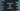# TypeScript program to add two numbers### TypeScript program to add two numbers:

This is a basic TypeScript program. In this program, we will learn how to add two numbers or how to find the sum of two numbers in TypeScript. We will write a new function that will take the numbers as its parameters and return the sum.

Let’s write down the program.

### TypeScript program to add two given numbers:

To write the program, you need to create one .ts file. Suppose, the name of the file is example.ts. Create the file and add the below code:

``````function getSum(first: number, second: number){
return first + second;
}

console.log(getSum(10, 11));
console.log(getSum(13, 31));
console.log(getSum(10.44, 115.11));``````

Here,

• getSum is a function that takes two numbers as its parameters. first and second are these parameters. It returns the sum of the first and second.

It is using the console.log statement to print the result of getSum for three different sets of variables.

To run this program, you have to compile the TypeScript file. Run the following command in a terminal:

``tsc example``

It will create the JavaScript file in the same folder. It will hold the below code:

``````"use strict";
function getSum(first, second) {
return first + second;
}
console.log(getSum(10, 11));
console.log(getSum(13, 31));
console.log(getSum(10.44, 115.11));``````

Once the file is created, you can run the code with node. It will print the values of the sum.

``````21
44
125.55``````

### Error for invalid values:

TypeScript will show errors for invalid parameters. The getSum method can take parameters of number types. But, if we try to pass any other parameters of different types, it will show error.

For example,

``````function getSum(first: number, second: number){
return first + second;
}

console.log(getSum('10', '11'));``````

For this example, we are calling the getSum method with two string values. It will throw this error:

``````Argument of type 'string' is not assignable to parameter of type 'number'.

6 console.log(getSum('10', '11'));
~~~~

Found 1 error.``````

As we have defined the type of the parameters of the function getSum as number. But since we are passing strings, it throws an error.

### TypeScript program to add numbers of different types:

If we have to handle parameters of different types, we have to use union types in TypeScript. For example, suppose we want to get the sum of two variables and these variables can be of number or string type, we can write a program as like below:

``````function getSum(first: number | string, second: number | string) {
if (typeof first === "string") {
first = Number(first);
}
if (typeof second === "string") {
second = Number(second);
}

return first + second;
}

console.log(getSum("10", "11"));
console.log(getSum(10, "11"));
console.log(getSum("10", 11));
console.log(getSum(10, 11));``````

Here, the function getSum is changed. The parameters first and second can have number or string types. If the type of first or second is string, it converts that value to a number and assigns that value to that variable. At the end of the program, it returns the sum of the parameter values.

We are using four different examples with different parameter types. It will work for all and print 21 for all of these examples.